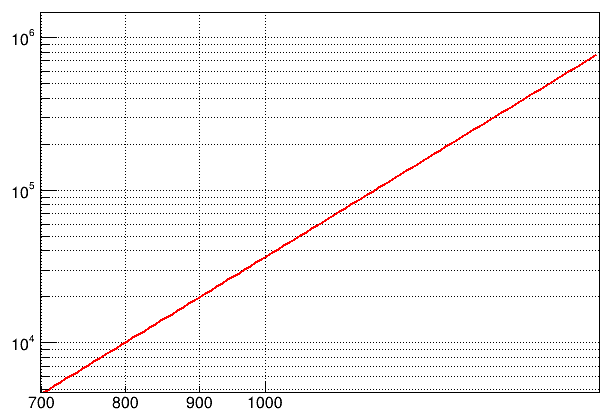# Log x axis ticks

Hello, I was wondering if there is a trick to add more ticks (as well as label and gridlines) at 1100, 1200, 1300, etc in the image below. The right area of the plot doesn’t look optimum, and I cannot figure out how to change it.``````    TCanvas* c = new TCanvas();
c->SetGridx();
c->SetGridy();
c->SetLogx();
c->SetLogy();
TF1* f = new TF1("f", "1.73E-13*pow(x, 5.775)", 699, 1701);
f->GetHistogram()->GetXaxis()->SetMoreLogLabels();
f->GetHistogram()->GetXaxis()->SetNoExponent();
f->Draw("");
``````

No, the next tick in log scale will be 2000

Thanks. Is there a way to manually add custom ticks and labels on top? Maybe with TLines?

Or is there a way to add secondary tick marks or line inbetween the current ones?

Based on this solution

You could do something like:

``````{
TCanvas* c = new TCanvas();
c->SetGridx();
c->SetGridy();
c->SetLogx();
c->SetLogy();
TLatex *t;
TLine *tick;
TF1* f = new TF1("f", "1.73E-13*pow(x, 5.775)", 699, 1701);
f->GetHistogram()->GetXaxis()->SetMoreLogLabels();
f->GetHistogram()->GetXaxis()->SetNoExponent();
f->Draw();
double ymin = f->GetHistogram()->GetMinimum();
double ymax = f->GetHistogram()->GetMaximum();
double dy = (ymax-ymin);
cout << "ymin/max " << ymin << " / " << ymax << endl;
for (int i=1; i<f->GetHistogram()->GetXaxis()->GetNbins();++i) {
double x = f->GetHistogram()->GetXaxis()->GetBinCenter(i);
double xL = f->GetHistogram()->GetXaxis()->GetBinLowEdge(i);
double xU = f->GetHistogram()->GetXaxis()->GetBinUpEdge(i);
if (xL<=1200 && xU>1200) {
t = new TLatex(x, 3770, Form("%d",1200));
t->SetTextSize(0.035);
t->SetTextFont(42);
t->SetTextAlign(21);
t->Draw();
tick = new TLine(x,ymin,x,ymin+0.0008*dy);
tick->Draw();
}
}
``````

where I determined the y of the TLatex (3770) and the factor for dy (0.0008) by trial and error, but you might find formulas for your case. From this you can surely figure out the vertical grid lines.

This topic was automatically closed 14 days after the last reply. New replies are no longer allowed.